International
Tables for
Crystallography
Volume B
Reciprocal space
Edited by U. Shmueli

International Tables for Crystallography (2006). Vol. B, ch. 5.2, p. 552   | 1 | 2 |

Section 5.2.3. Forward scattering

A. F. Moodie,a J. M. Cowleyb and P. Goodmanc

aDepartment of Applied Physics, Royal Melbourne Institute of Technology, 124 La Trobe Street, Melbourne, Victoria 3000, Australia,bArizona State University, Box 871504, Department of Physics and Astronomy, Tempe, AZ 85287-1504, USA, and cSchool of Physics, University of Melbourne, Parkville, Australia 3052

5.2.3. Forward scattering

| top | pdf |

A great deal of geometric detail can arise at this point and, further, there is no generally accepted method for approximation, the various procedures leading to numerically negligible differences and to expressions of precisely the same form. Detailed descriptions of the geometry are given in the references.

The entrance surface of the specimen, in the form of a plate, is chosen as the x, y plane, and the direction of the incident beam is taken to be close to the z axis. Components of the wavevector are labelled with suffixes in the conventional way;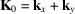is the transverse wavevector, which will be very small compared to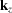. In this notation, the excitation error for the reflection is given by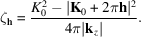An intuitive method argues that, since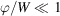, then the component of the motion along z is little changed by scattering. Hence, making the substitution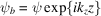and neglecting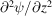, equation (5.2.2.1)becomeswhere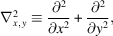and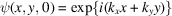.

Equation (5.2.3.1)is of the form of a two-dimensional time-dependent Schrödinger equation, with the z coordinate replacing time. This form has been extensively discussed. For instance, Howie (1966) derived what is essentially this equation using an expansion in Bloch waves, Berry (1971) used a Green function in a detailed and rigorous derivation, and Goodman & Moodie (1974), using methods due to Feynman, derived the equation as the limit of the multislice recurrence relation. A method due to Corones et al. (1982) brings out the relationship between the HEED and LEED equations. Equation (5.2.2.1)is cast in the form of a first-order system,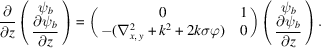A splitting matrix is introduced to separate the wavefunction into the forward and backward components,, and the fast part of the phase is factored out, so that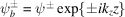. In the resulting matrix differential equation, the off-diagonal terms are seen to be small for fast electrons, and equation (5.2.2.1)reduces to the pair of equations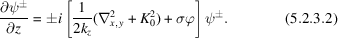The equation for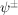is the Lontovich & Fock (1946) parabolic equation.

References

Berry, M. V. (1971). Diffraction in crystals at high voltages. J. Phys. C, 4, 697–722.Google Scholar
Corones, J., De Facio, B. & Kreuger, R. J. (1982). Parabolic approximations to the time-independent elastic wave equation. J. Math. Phys. 23, 577–586.Google Scholar
Goodman, P. & Moodie, A. F. (1974). Numerical evaluation of N-beam wave functions in electron scattering by the multislice method. Acta Cryst. A30, 280–290.Google Scholar
Howie, A. (1966). Diffraction channelling of fast electrons and positrons in crystals. Philos. Mag. 14, 223–237.Google Scholar
Lontovitch, M. & Fock, R. (1946). Solution of the problem of propagation of electromagnetic waves along the Earth's surface by the method of parabolic equation. J. Phys. 10, 13–24. (Translated from Russian by J. Smorodinsky.)Google Scholar